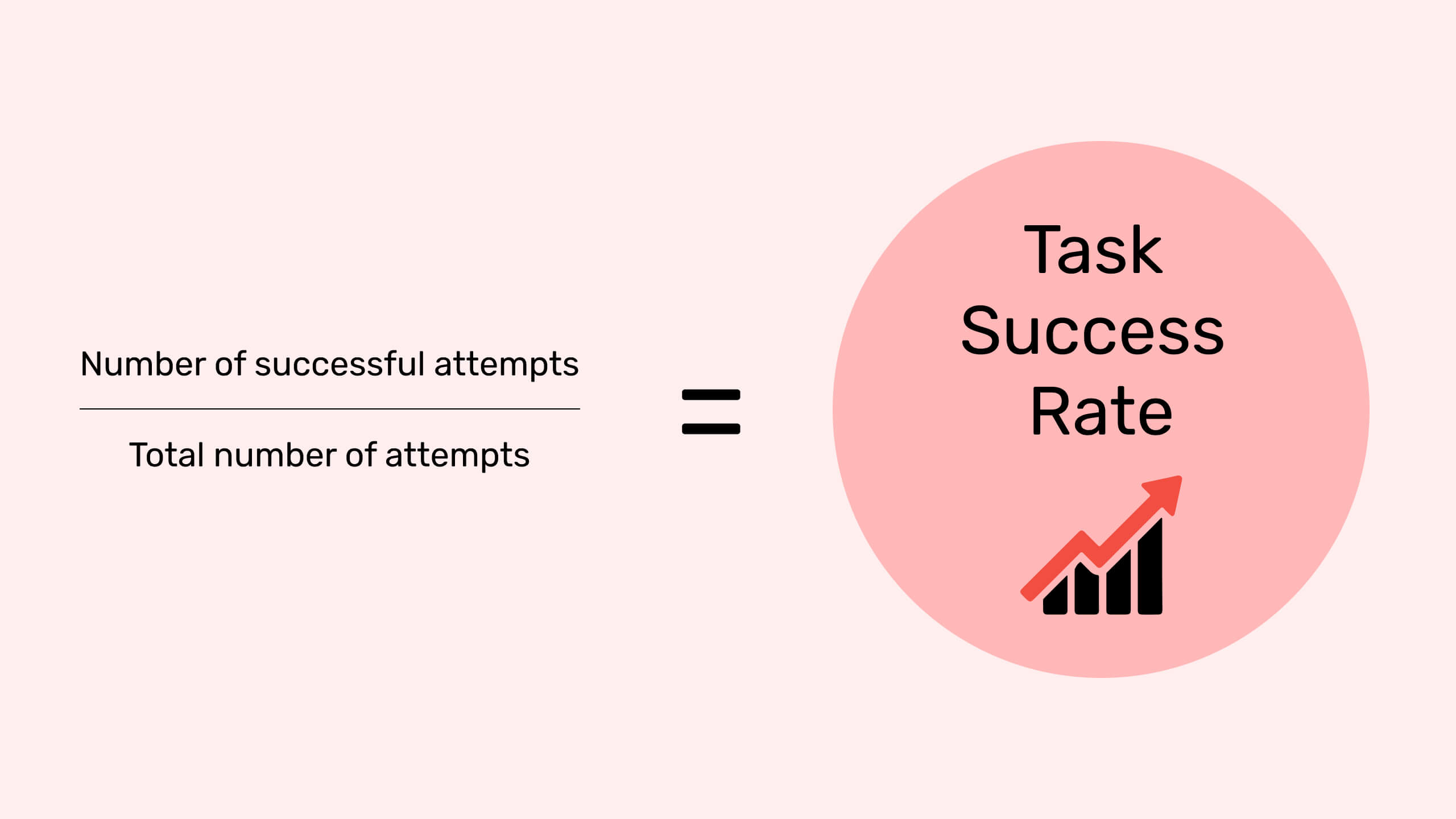category: UIUX 360

# Error RateScroll Down## What is Error Rate?

### Overview

The error rate is numbers or frequency or other proportion of errors; relative to correct task completions for an interface. Errors are when a user fails to perform a task or step. EG: If a task has 5 possibilities of error and 10 users try to do the task then there are 50 possibilities. If you see 5 errors in users the error rate is 5/50 = 10%.

Errors are a common occurrence in usability testing and result in problems with the interface and incomplete human actions. A product’s error rate refers to how inaccurate the model's predictions are.

With the help of the error rate easily calculate the ratio of the job completion rate. For example, if a user made 3 errors for 1 task you can't just divide 3/1.

For instance, if the predicted value is assumed to be binary (is_male=True/False), the error rate would be as the percentage of incorrect answers. Each time the model guessed the male when the correct/true value was female or vice versa.### Boost your design scope with Think 360

Book a call with us and get the party started!